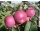55%+36%+88%+71%+100=63%
what is whole (X)?

Percents can be added directly together if they are taken from the same whole, which means they have the same base amount. .. . You would add the two percentages to find the total amount.

Result

X =  18

#### Solution:

0.55X+0.36X+0.88X+0.71X+X= 63

3.5X = 63

X = 18

Calculated by our simple equation calculator.

Leave us a comment of example and its solution (i.e. if it is still somewhat unclear...):Be the first to comment!#### To solve this example are needed these knowledge from mathematics:

Our percentage calculator will help you quickly calculate various typical tasks with percentages. Do you have a linear equation or system of equations and looking for its solution? Or do you have quadratic equation?

## Next similar examples:

1. Jane classWhen asked how many students are in class, Jane said, if we increase the number of students in our class by hundred % and then add half the number of students, we get 100. How many students are in Jane's class?
2. CompetitorsIn the first round of slalom fell 15% of all competitors and in the second round another 10 racers. Together, 40% of all competitors fell. What was the total number of competitors?
3. Perctentages35% of what number is 35?
4. Profit gainIf 5% more is gained by selling an article for Rs. 350 than by selling it for Rs. 340, the cost of the article is:
5. EmployeesThere are 1116 people working in three factory halls. In the first one, there are 18% more than the third, and 60 persons more than the second. How many employees work in individual halls?
6. Company and employeesThere are 370 employees in the company - women are 15% less than men. How many men work in the company?
7. Commission 2Mr gomez sells used cellphones. His commission for every cellphone sold is 20%. If his total sales is Php 33850, how much is his commission? Please, please, please show your solution.
8. PineHow much of a mixed forest rangers want to cut down, if their head said: "We only cut down pine trees, which in our mixed forest 98%. After cut pine trees constitute 94% of all trees left."
9. BorrowingI borrow 25,000 to 6.9% p.a.. I pay 500 per month. How much will I pay and for how long?
10. ThereThere are 32 trees of three species in the orchard. 25% cherries, three eighty pears, others plums. How many plums are in the orchard?
11. Cinema ticketsCinema sold 180 tickets this Thursday, which is 20%. Monday 14%, Tuesday 6%, Wednesday 9%, Friday 24%, Saturday 12%, and Sunday 15%. How many tickets were sold per week?
12. Reducing numberReducing the an unknown number by 28.5% we get number 243.1. Determine unknown number.
13. PupilsThere are 350 girls in the school, and the other 30% of the total number of pupils are boys. How many pupils does the school have?
14. PercentCalculate how many % is the number 26.25 less than the number 105.
15. Theorem proveWe want to prove the sentence: If the natural number n is divisible by six, then n is divisible by three. From what assumption we started?
16. ChildrenThe group has 42 children. There are 4 more boys than girls. How many boys and girls are in the group?
17. EquationHow many real roots has equation ? ?